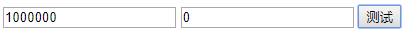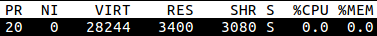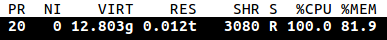# 各种编程语言对尾递归的支持

`　　版权申明：本文为博主窗户(Colin Cai)原创，欢迎转帖。如要转贴，必须注明原文网址    　　http://www.cnblogs.com/Colin-Cai/p/11774213.html    　　作者：窗户    　　QQ/微信：6679072    　　E-mail：[email protected]`

尾递归

这篇文章，我们讲尾递归。在递归中，如果该函数的递归形式表现在函数返回的时候，则称之为尾递归。

举个简单的例子，用伪码如下：

if a = 0

return b

end

上述代码很容易用循环表示：

while True

if a = 0

return b

end

a <= a-1

b <= b+1

end

end

所有的尾递归都可以用循环表示，只需要把传入的参数当成是状态，运算的过程当成是状态的转换。

3,0

2,1

1,2

0,3

这样的状态转换。

函数的计算会维护一个栈，每当遇到函数调用会记录当前运行的状态，如此在函数返回的时候可以恢复上下文。

比如，对于Fibonacci数列，伪码如下：

function fib(n)

if n < 3

return 1

end

return fib(n-1)+fib(n-2)

end

我们计算fib(4)，栈大致如下：

fib(4)

=>

fib(4)

fib(3)

=>

fib(4)

fib(3)

fib(2)

=>

fib(4)

fib(3)

fib(2) 1

=>

f(4)

f(3) 1+

=>

f(4)

f(3) 1+

f(1)

=>

f(4)

f(3) 1+

f(1) 1

=>

f(4)

f(3) 2

=>

f(4) 2+

=>

f(4) 2+

f(2)

=>

f(4) 2+

f(2) 1

=>

f(4) 3

=>

3

=>

=>

=>

=>

=>

=>

=>

=>

3

这里，可以采用一个编译技术，就是尾递归优化，其一般情况是，如果一个函数的计算中遇到了完全转化成另一个函数调用的情况，那么栈的当前函数部分的信息可以完全抹去，而替换为新的函数。如此处理下，此情况栈不会增长。

=>

=>

=>

=>

3

尾递归优化给了我们一种迭代的方式，之所以研究它，在于函数式编程会用到它。

注：递归论区分递归和迭代(迭置)，和计算机上定义有一点区别，在此不深入。

C/C++

我们从底层的语言开始，首先还是上面的加法实现。为了让范围更大一点，便于观察，我们使用unsigned long long类型。

`/*add.c*/  unsigned long long add(unsigned long long a, unsigned long long b)  {          if(a==0ULL)                  return b;          return add(a-1ULL,b+1ULL);  }`

`#include <stdio.h>  unsigned long long add(unsigned long long a, unsigned long long b);  int main(int argc, char **argv)  {      unsigned long long a, b;      sscanf(argv, "%llu", &a);      sscanf(argv, "%llu", &b);      printf("%llun", add(a,b));      return 0;  }`

用gcc编译，

运行一下，

./a.out 10000000 100000000

马上发生短错误，直接崩溃。看来C语言作为底层语言没必要支持这个啊？

于是我们开启优化，

gcc -O2 add.c main.c -o a.out

然后运行一下

./a.out 10000000000000000 10000000000000000

立即得到我们想要的值而没有发生崩栈

20000000000000000

看来……不对，1亿亿次迭代瞬间完成？

objdump反汇编一把，

`00000000004006b0 <add>:    4006b0:       48 8d 04 37             lea    (%rdi,%rsi,1),%rax    4006b4:       c3                      retq   `

……原来全被优化了，gcc现在还真强大，直接猜出语义，clang测一把也是如此。

这个并非我们想要的，我们得用其他手段去验证(其实我们可以抽出部分优化选项来，但此处讲的是验证思路)。

此处借助我在《相互递归》中讲的奇偶判断，分三个函数，实现如下，

`/*sub1.c*/  unsigned long long sub1(unsigned long long x)  {          return x - 1ULL;  }`

`/*is_odd.c*/  unsigned long long sub1(unsigned long long x);  int is_even(unsigned long long x);  int is_odd(unsigned long long x)  {          if(x == 0ULL)                  return 0;          return is_even(sub1(x));  }`

`/*is_even.c*/  unsigned long long sub1(unsigned long long x);  int is_odd(unsigned long long x);  int is_even(unsigned long long x)  {          if(x == 0ULL)                  return 1;          return is_odd(sub1(x));  }`

上述函数是单独编写，甚至，减1的操作也单独用一个文件来实现。如此测试的原因，就在于，我们要排除掉整体优化的可能。

还需要写一个main函数来验证，

`/*main.c*/  #include <stdio.h>  int is_odd(unsigned long long x);  int main(int argc, char **argv)  {      unsigned long long x;      sscanf(argv, "%llu", &x);      printf("%llu is %sn", x, is_odd(x)?"odd":"even");      return 0;  }`

以上四个文件单独编译，开启-O2优化选项(当然，其实main无所谓)

for i in sub1.c is_odd.c is_even.c main.c; do gcc -O2 -c \$i; done

然后链接，

gcc sub1.o is_odd.o is_even.o main.o -o a.out

然后我们对一个很大的数来进行测试，

./a.out 10000000000

一会儿之后，程序打印出

10000000000 is even

以上可以证明，gcc/clang对于尾递归优化支持的挺好。实际上，很早之前大部分C语言编译器就支持了这点，因为从技术上来看，并不是很复杂的事情。而C++也同理。

Python

`def add(a, b):      if a==0:          return b      return add(a-1, b+1)`

不过这里栈似乎小了点，可以用sys.setrlimit来修改栈的大小，这实际上是UNIX-like的系统调用。

有人用捕捉异常的方式让其强行支持尾递归，效率当然是损失很多的，不过这个想法倒是很好。想起以前RISC大多不支持奇边界存取值，比如ARM，于是在内核中用中断处理强行支持奇边界错误，虽然效率低了很多，但逻辑上是通过的。异曲同工，的确也是一条路，不过我还是更加期望Python在未来支持尾递归优化吧。

JavaScript

`<input type="text" id="in1" />  <input type="text" id="in2" />  <input type="button" id="bt1" onclick="test()" value="测试"/>  <script type="text/javascript">  function add(a, b)  {      if (a==0) {          return b;      }      return add(a-1, b+1);  }    function test()  {      a = parseInt(document.getElementById("in1").value);      b = parseInt(document.getElementById("in2").value);      try {          alert(add(a,b));      }      catch(err) {          alert('Error');      }  }  </script>`就用1000000和0来测试，没看到哪个浏览器不跳出Error的……据说v8引擎做好了，可是人家就不给你用……

Scheme

然后我们来看Scheme，按照Scheme的标准一向强行规定Scheme支持尾递归优化。

`(define (add a b)   (if (zero? a) b (add (- a 1) (+ b 1))))`

实现更为复杂的奇偶判断

`(define (is-odd x)      (if (zero? x) #f (is_even (- x 1))))  (define (is-even x)      (if (zero? x) #t (is_odd (- x 1))))`

使用Chez Scheme、Racket、guile测试，使用很大的数来运算，

然后使用top来观测程序的内存使用情况，我们发现，虽然CPU占用率可能是100%，但内存的使用并不增加。就连guile这样的一个小的实现都是如此，从而它们都是符合标准而对尾递归进行优化的。

Common Lisp

测完Scheme，再来测Scheme的本家兄弟，另外一种Lisp——Common Lisp

`(defun add(a b)   (if (zerop a) b (funcall #'add (- a 1) (+ b 1))))`

使用clisp来运行

结果就

*** – Program stack overflow. RESET

`(defun add(a b)   (do    ((x a (- x 1))     (y b (+ y 1)))    ((zerop x)  y)))`

如此，终于不崩栈了。但是似乎也改变了Lisp的味道，do显然此处只能在设计编译器、解释器的时候就得单独实现，虽然按理Lisp下这些都应该是宏，但是无论用宏如何将函数式编程映射为显示的迭代，因为尾clisp递归优化不支持，则无法和系统提供的do一样。

`(defun is-odd(x)   (if (zerop x) '() (funcall #'is-even (- x 1))))  (defun is-even(x)   (if (zerop x)  t (funcall #'is-odd (- x 1))))`

计算

（is-even 1000000000)

过了几秒，返回了结果t，证明了sbcl对尾递归做了优化。也终于给了我们一个更为靠谱的Common Lisp的实现。

AWK

`awk '  function add(a,b)  {     if(a==0)         return b      return add(a-1, b+1)  }  {print add(\$1, \$2)}'`

运行后，用top来观测内存占用输入

100000000 1

让其做加法内存使用瞬间爆发，直到进程被系统KO。

话说，awk没有对尾递归优化也属正常，而且对于内存的使用还真不节制，超过了我的想象。不过这也与语言的目的有关，awk本就没打算做这类事情。

`is_even x = if x==0 then True else is_odd (x-1)  is_odd x = if x==0 then False else is_even (x-1)  main = print (is_even 1000000000)`

用ghc编译运行，输出True，用时33秒。

Prolog

本不想测prolog，因为首先它并没有所谓的函数，靠的是谓词演化来计算，推理上的优化是其基本需求。尾递归本不属于Prolog的支持范畴，当然可以构造类似尾递归的东西，而且Prolog当然可以完成，不会有悬念。

比如我们实现奇偶判断如下：

`is_even(0, 1).  is_even(X, T) :- M is X-1, is_odd(M, T).  is_odd(0, 0).  is_odd(X, T) :- M is X-1, is_even(M, T).`

查询

?- is_even(100000000,S),write(S),!.

得到

1

Erlang

`-module(mytest).  -export([add/2,even/1,odd/1]).  add(A,B)->if A==0->B;true->add(A-1,B+1) end.  even(X)->if X==0->true;true->odd(X-1) end.  odd(X)->if X==0->false;true->even(X-1) end.`

加载模板，并测试如下

1> c(mytest).
{ok,mytest}
2000000000
3> mytest:even(1000000000).
true
4> mytest:odd(1000000000).
false

显然，Erlang对尾递归支持很好。

golang

`package main  import "fmt"  func add(a int, b int) int {      if (a==0) {          return b;      }      return add(a-1,b+1);  }    func main() {      fmt.Println(add(100000000, 0))  }`

运行

马上崩溃

Lua

Lua的作者和JS的作者一样是Lisp的粉丝，Lua的后期设计(从Lua4)据说参考了Scheme。

`function odd(x)      if (x==0) then          return false      end      return even(x-1)  end  function even(x)      if (x==0) then          return true      end      return odd(x-1)  end  print(odd(io.read()))`

运行

echo 1000000000 | lua5.3 x.lua

过程中，观察内存没有明显变化，之后打印出了false

看来，至少参考了Scheme的尾递归优化。

Ruby

Ruby的作者松本行弘也是Lisp的粉丝，当然，我想大多数编程语言的作者都会是Lisp的粉丝，因为它会给人很多启发。

实现奇偶判断如下：

`#!/usr/bin/ruby    def odd(x)      if x == 0          return 0      end      return even(x-1)  end    def even(x)      if x == 0          return 1      end      return odd(x-1)  end    puts even gets.to_i`

然而，数字大一点点，就崩栈了。Ruby并不支持尾递归优化。

测了这些语言以及相应的工具，其实还是在于函数式编程里，尾递归实现的迭代是我们经常使用的手段，编译器/解释器的支持就会显得很重要了。再深一步，我们会去想想，编译器/解释器此处该如何做，是否可以对现有的设计进行修改呢？或者，对该语言/工具的未来怀着什么样的期待呢？再或者，如果我们自己也设计一种编程语言，会如何设计这种编程语言呢？……